# Moles to Grams Conversion Formula

Moles to Grams Conversion Formula

In order to convert the moles of a substance to grams, you will need to multiply the mole value of the substance by its molar mass.More commonly written for this application as:where,is the molar mass of the substance.

Moles to Grams Conversion Formula Questions:

1. How many grams are in 3.79 moles of calcium bromide, CaBr2?

Answer:

First, you will need to calculate the molar mass of calcium bromide by using the periodic table and the number of each element in the formula.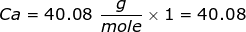Now, use the number of moles and multiply it by the molar mass.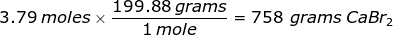2. How many grams are in 0.572 moles of glucose, C6H12O6?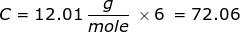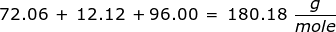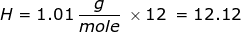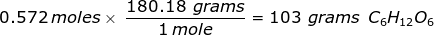Related Links: